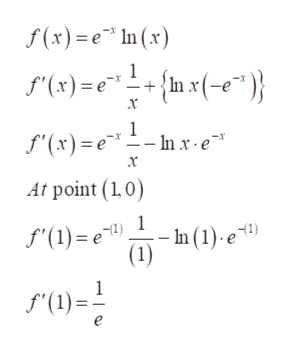# Find an equation of the tangent line to the graph of the function at the given point.f(x) = e−x ln(x),    (1, 0)

Question
2 views

Find an equation of the tangent line to the graph of the function at the given point.

f(x) = ex ln(x),    (1, 0)
check_circle

Step 1

Refer to the question we need to find an equation of the tangent line to the graph of the provided function at the given point.

Step 2

Use the product rule of derivative as,help_outlineImage Transcriptioncloseuf(x)v=g(x) (n)p dx dv)d(u) Product rule: f'(uv) =u- dx d(e) dx d(In x) 1 dx fullscreen
Step 3

To find the equation of tangent line to the graph fin...help_outlineImage Transcriptionclosef(x)e In (x) {n x(-e")} 1 f'(x)e (x)=nx) '(x)= -In x e х At point (1,0) 1 f'(1) e) hn (1).е 1) f(1) fullscreen

### Want to see the full answer?

See Solution

#### Want to see this answer and more?

Solutions are written by subject experts who are available 24/7. Questions are typically answered within 1 hour.*

See Solution
*Response times may vary by subject and question.
Tagged in

### Calculus